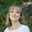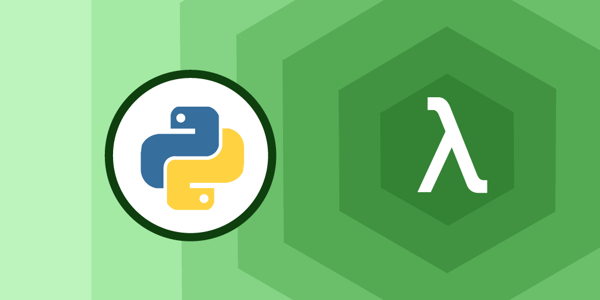# How to use Python Lambda functions: a 5 minute tutorial

Dec 02, 2020 - 6 min readAmanda FawcettPython has many features for implementing functional programming concepts. When writing functional-style programs, you often need small functions that combine elements. Python has a built-in way to do this using lambda functions.

In computer programming, an anonymous function (such as a lambda expression) is a function not bound to an identifier. Lambda functions are an important part of functional programming that allow you to write throw-away functions without needing to name them.

In this Python tutorial, we will introduce you to lambda functions in Python and show you how to implement them in your own code.

Today, we will learn:

## What are lambda functions?

A lambda function is a small, anonymous function that take any number of arguments but only have one expression. Lambda functions return an object that is assigned to a variable or used as a part of other functions.

Lambdas differ from regular function definitions in several ways. Most notably, lambda functions are restricted to a single expression, so they can’t use statements or annotations.

When it comes to return values from lambdas, there is always an implicit return statement. Lambda functions evaluate the expression and automatically return a result.

This is why some programmers call lambdas “single expression functions”.

A lambda function does not need a name during function definition, unlike a normal function. We create them with the lambda keyword instead of the traditional def keyword. The structure of lambda can be seen below:History: Lambda expressions comes from concepts in lambda calculus, a model of computation invented by Alonzo Church.

Though Python is not fully a functional language, it has added many functional concepts. In 1994, filter(), map(), reduce(), and the lambda operator were added to its syntax. Other object-oriented programming languages like Java and JavaScript also added lambda expressions in later versions.

## When to use lambda functions

Lambda functions have dozens of use cases, but they are most commonly used when function objects are required. The beauty of lambda functions is that they return function objects.

This makes them helpful when used alongside higher-order functions that require function objects as arguments, such as map(), filter(), or functools.reduce()

It is a best practice to use lambdas when the function expression is small to help with readability. It’s a good idea to use lambda functions when it provides the shortest way to write or compute something, for example, when:

• Returning a function from a function
• Sorting by an alternate key
• Combining elements of an iterable sequence with reduce()

Once you get used to lambda expressions, you’ll start using them quite often. They are expressive and make code shorter and more readable when used properly. To make the most of lambda, follow these general guidelines:

• Lambdas can only contain a single expression. If your function cannot be expressed in one line, don’t use a lambda.
• It is best to use them only for short and simple code, where the behavior of the function is obvious.
• If a function call uses several lambda expressions, it might be difficult to see what is going on, and it is not recommended.
• If the same function is used in several places, it is usually better to define a normal function rather than repeating the lambda.

## Keep the learning going.

Learn Lambda expressions in Python without scrubbing through videos or documentation. Educative’s text-based courses are easy to skim and feature live coding environments - making learning quick and efficient.

Learn Functional Programming in Python

## How to implement Python lambda functions

A lambda function is declared differently than a normal function. In Python, lambda functions have the following unique characteristics:

• It can only contain expressions
• It cannot include statements in its body
• It is written as a single line
• It does not support type annotations
• It can be immediately invoked

A lambda function in Python uses the following basic syntax. As we mentioned before, we use the lambda keyword to create a simple function in a Python expression.

lambda arguments: expression


A lambda expression can have any number of arguments (including none). For example:

lambda: 1 # No arguments
lambda x, y: x + y
lambda a, b, c, d: a*b + c*d


In the following example, we use a lambda function to replace an area function:

lambda x: x*x


The lambda keyword identifies the lambda expression. In the above example, x is the only parameter. The colon ends the parameter list and introduces the body of the function.

To use this expression properly, you place it wherever you might normally use a function object. This piece of code below creates a temporary, anonymous function object and passes it into the sorted function, which then performs the sort.

p = [(3, 3), (4, 2), (2, 2), (5, 2), (1, 7)]

q = sorted(p, key=lambda x: x*x)
print(q) # [(2, 2), (1, 7), (4, 2), (3, 3), (5, 2)] 
Passing a lambda function as the value to the key parameter of the sorted function

Note: Lambda functions do not have names, but if you really want to, you can assign it to a variable, like below:

area = lambda x: x*x


There is no overt benefit to adding a function name to a lambda function.

## Python lambda examples

Now that we understand how lambda functions work in Python, let’s solidify our knowledge with some more examples and use cases.

### A squaring function

# A squaring lambda function
square = lambda n : n*n
num = square(5)
print num

### A subtraction function

# A subtraction lambda function with multiple arguments
sub = lambda x, y : x-y
print(sub(5, 3))

### Map with lambda

A Python lambda makes the map function far more concise.

myList = [10, 25, 17, 9, 30, -5]
# Double the value of each element
myList2 = map(lambda n : n*2, myList)
print myList2

### Filter with lambda

Lambdas can also simplify the filter() function.

myList = [10, 25, 17, 9, 30, -5]
# Filters the elements which are not multiples of 5
myList2 = filter(lambda n : n%5 == 0, myList)
print myList2

## Try it yourself

Let’s apply what we’ve learned so far to two hands-on examples. First, write a chunk of code using a lambda expression that adds 10 to argument a and returns the result. You can select any value for a. Try it yourself before checking the solution.

x = # type your code here
print # type your code here

For another activity, use lambda to multiply a with argument b and return the result. You can choose ant numbers to multiply when you print. Try it yourself before checking the solution.

x = # write your code here
print # write your code here

## What to learn next

Congrats! You should now have a solid understanding of lambda expressions in Python. You can can start implementing them in your own code. There is still more to learn when it comes to Python functional programming! Next you should check out:

• Higher-order functions with lambda
• The functools module
• Decorators and closures
• Aliases in Python
• and more

To get started with functional programming in Python, check out Educative’s course Learn Functional Programming in Python. In this course, you’ll learn what functional programming is, how it’s used in Python. You will everything from lambda to perform recursion to generators, and more.

By the end, you’ll have the confidence to use functional programming in your projects.

Happy learning!